### Choose language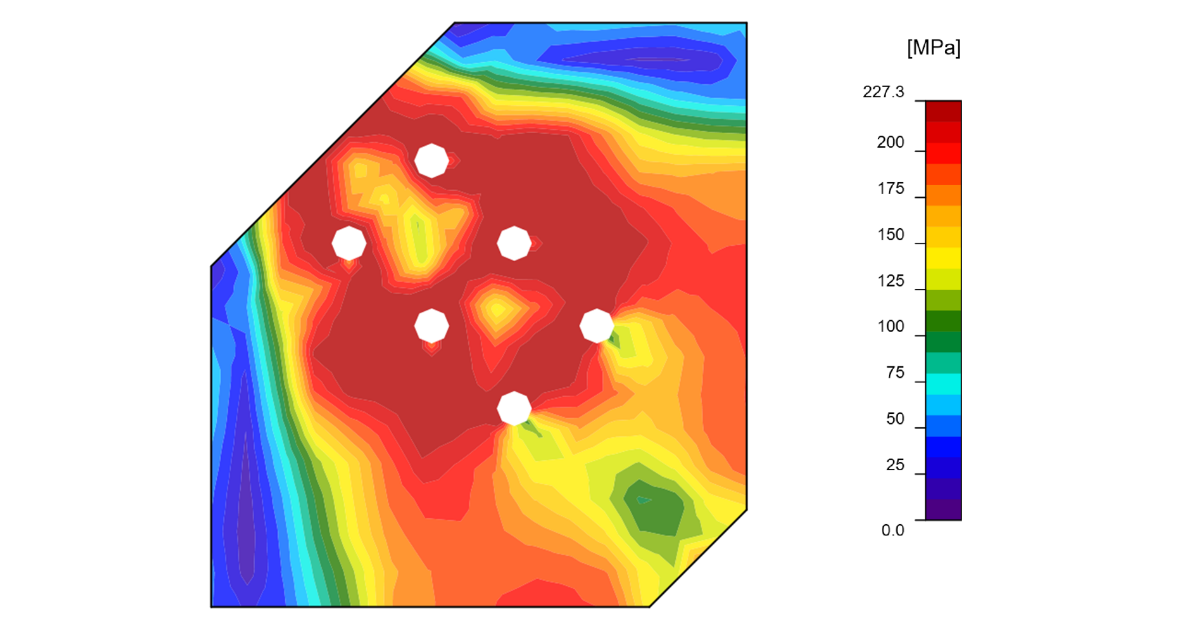# Block shear resistance

A study on the comparison of Block Shear Resistance as per IS code is done to validate the results of IDEA StatiCa Connection design software.
$$Type of Connection: Gusset PlateUnit System: MetricDesign Code: IS 800Plate Material: IS 2062 Grade SteelBolt: M16, Grade 8.8Geometry: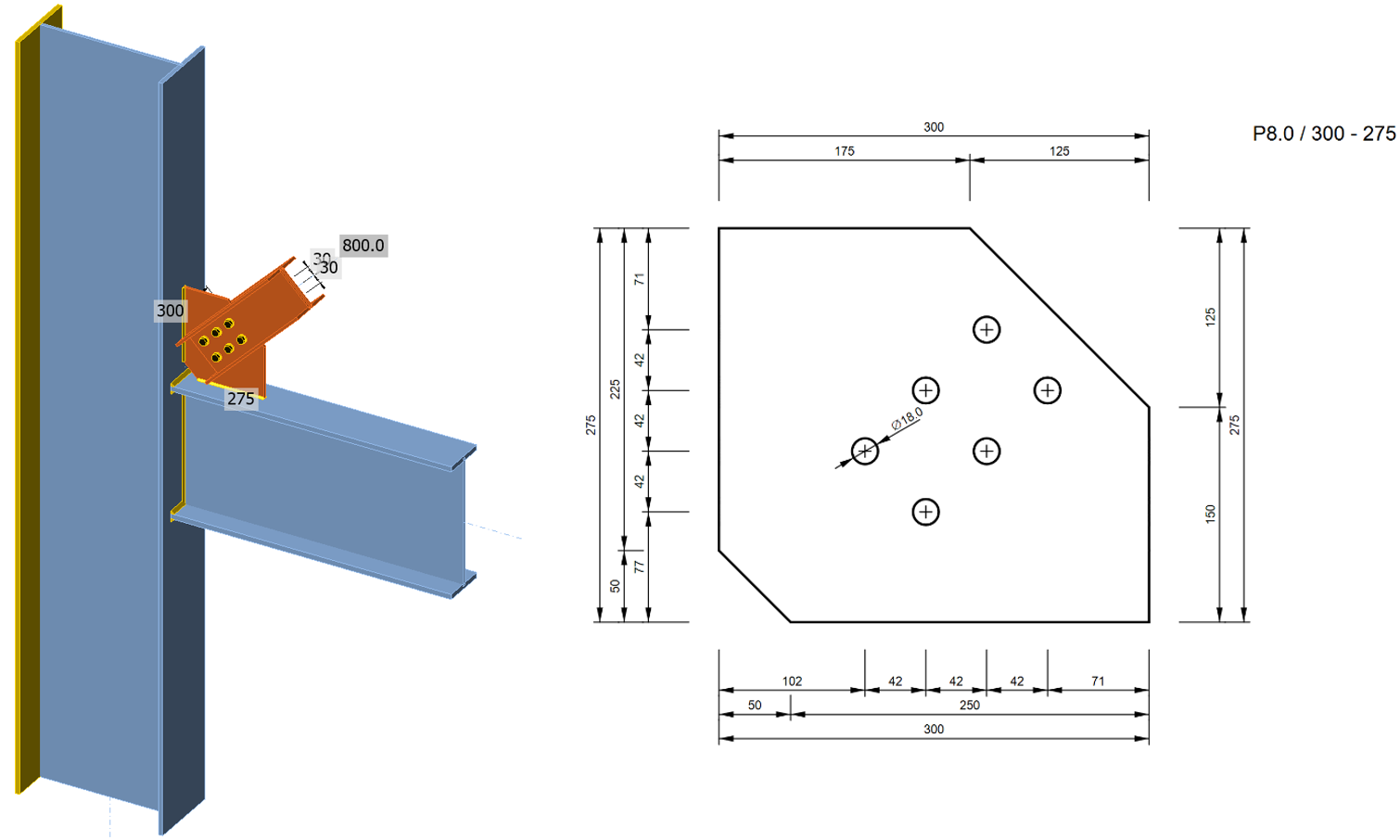Applied Forces:N = 800 kNV = 0 kNM = 0 kNProcedure:Block shear resistance is checked on a thin gusset plate (thickness 8 mm), which is designed to fail while other components are still undamagedIS Code: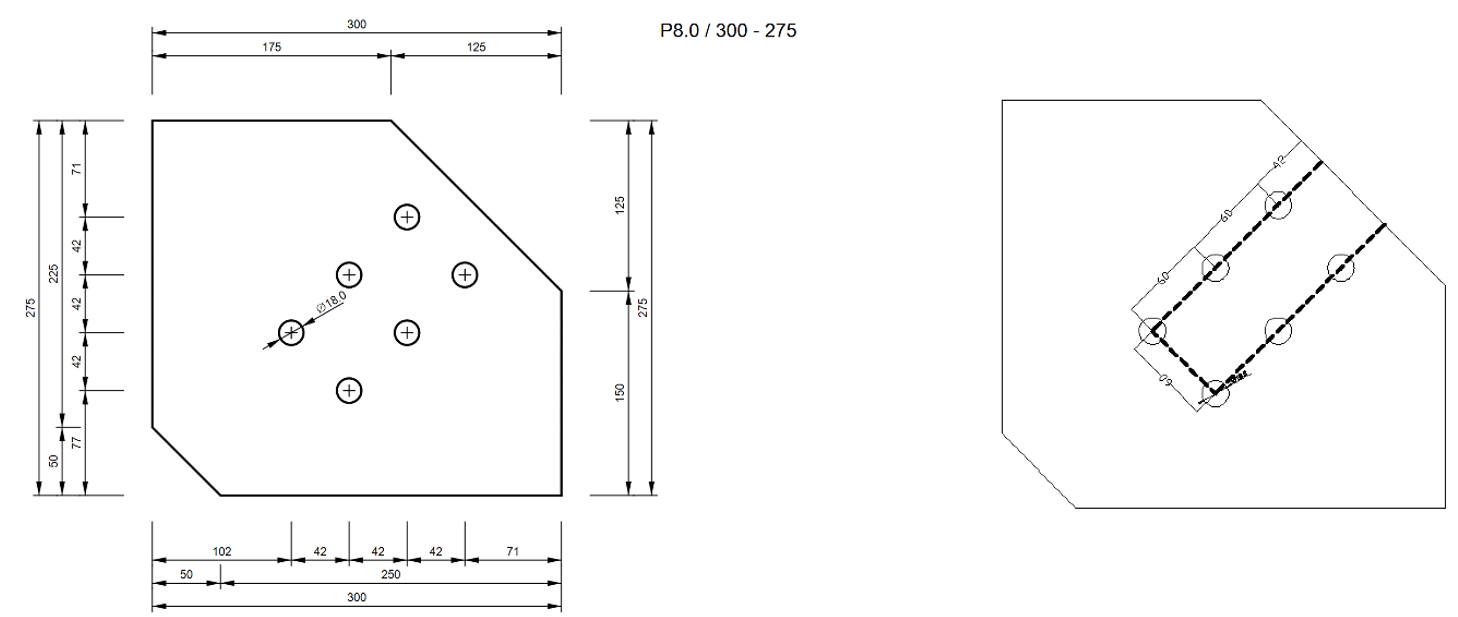Fig1. Gusset plate dimenions and failure of plate in block shearAs per Clause 6.4.1 of IS 800: 2007, the block shear strength (Tdb) of connection shall be taken as the smaller of$T_{db} = \frac{A_{vg} f_y}{\sqrt{3} \gamma_{m0}} + \frac{0.9 A_{tn} f_u}{\gamma_{m1}}$or$T_{db} = \frac{0.9 A_{vn} f_u}{\sqrt{3} \gamma_{m1}} + \frac{A_{tg} f_y}{\gamma_{m0}}$where: Avg, Avn = minimum gross and net area in shear along bolt line parallel to external force Atg, Atn = minimum gross and net area in tension from the bolt hole to the toe of the angle, end bolt line, perpendicular to the line of force fu, fy = ultimate and yield stress of the material Manual Calculations:Avg = (60+60+42) *8*2 = 2592 mm2Avn = ((60+60+42) – 2.5*18) *8*2 = 1872 mm2Atg = (60) *8 = 480 mm2Atn = (60-18) *8 = 336 mm2IDEA StatiCa Connection:The resistance of plate in IDEA is determined at 5% limit strain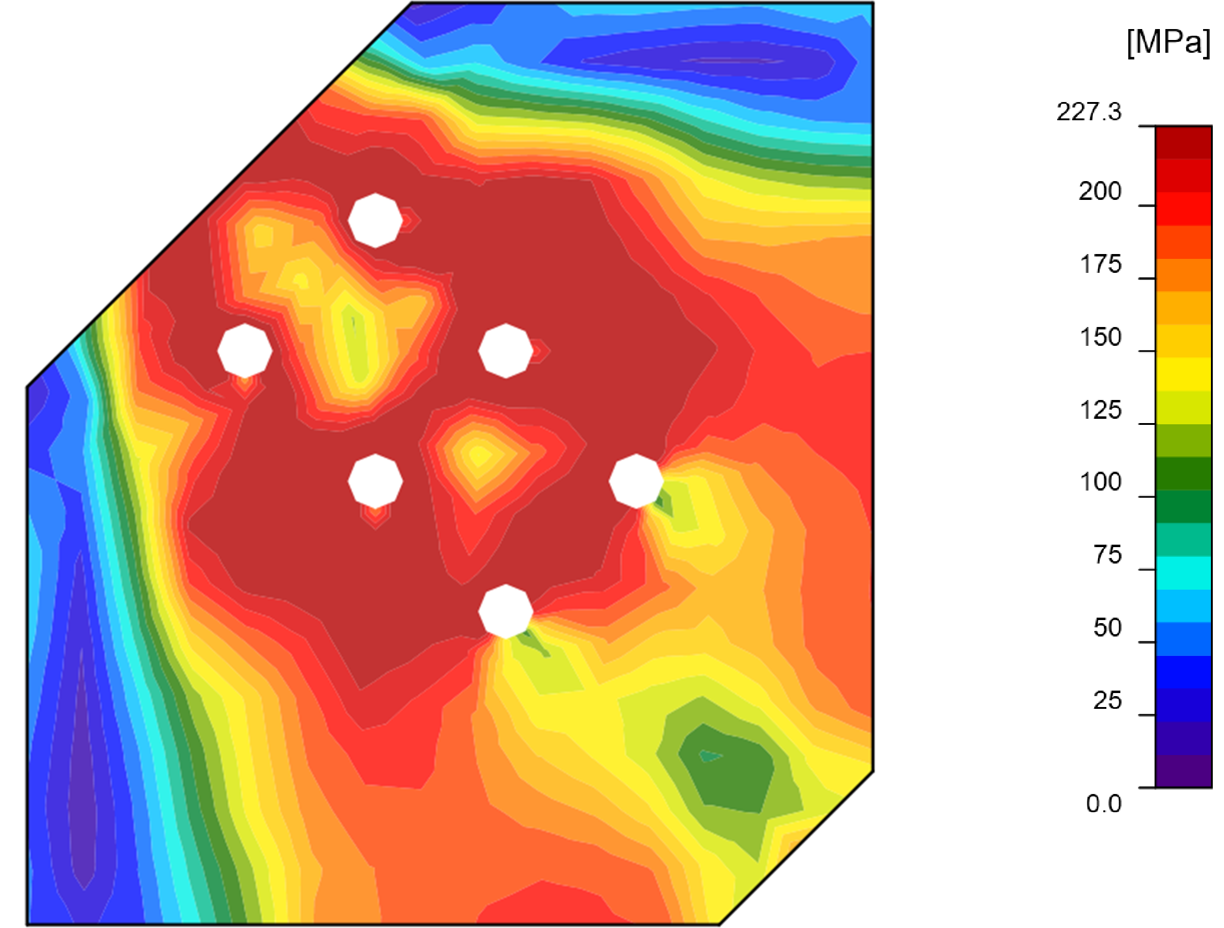Fig 2: Equivalent Stress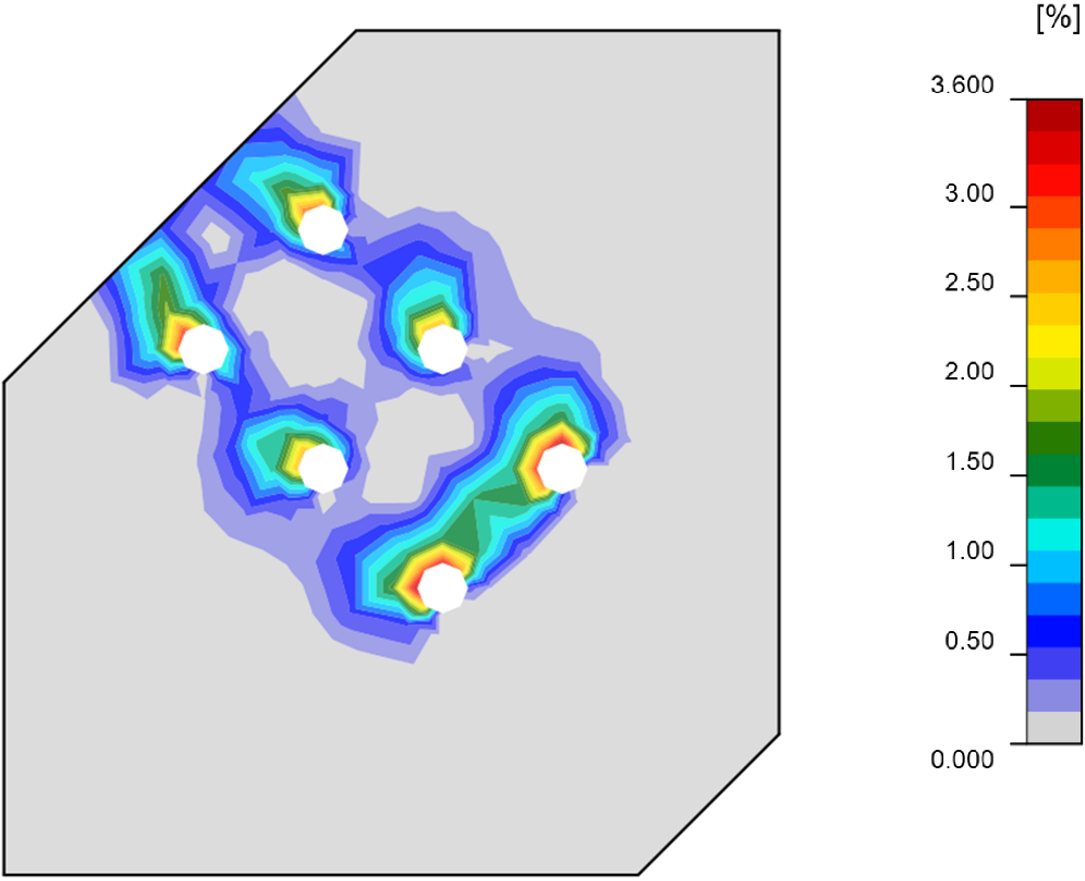Fig 3: Limiting StrainComparison: Steel Gradefy [MPa] fu [MPa] Tdb [kN] IS 800 Tdb [kN] IDEA IDEA/IS 800 E 165 (Fe 290)16529029527292% E 250 (FE 410 W) B25041042840394% E 300 (Fe 440)440300473472100% E 350 (Fe 490)490350534544102% E 410 (Fe 540)540410599628105% E 450 (Fe 570) D450570640669105% E 450 (Fe 590) E450590655669102% IDEA StatiCa Connection design results of block shear resistance are similar to manual computation according to IS 800: 2007. IDEA StatiCa Connection results are a little bit on the conservative side for low-grade steels and slightly unconservative (5 % at most) for high-grade steels. The reason is that the ultimate strength does not affect the results of IDEA Connection for plates.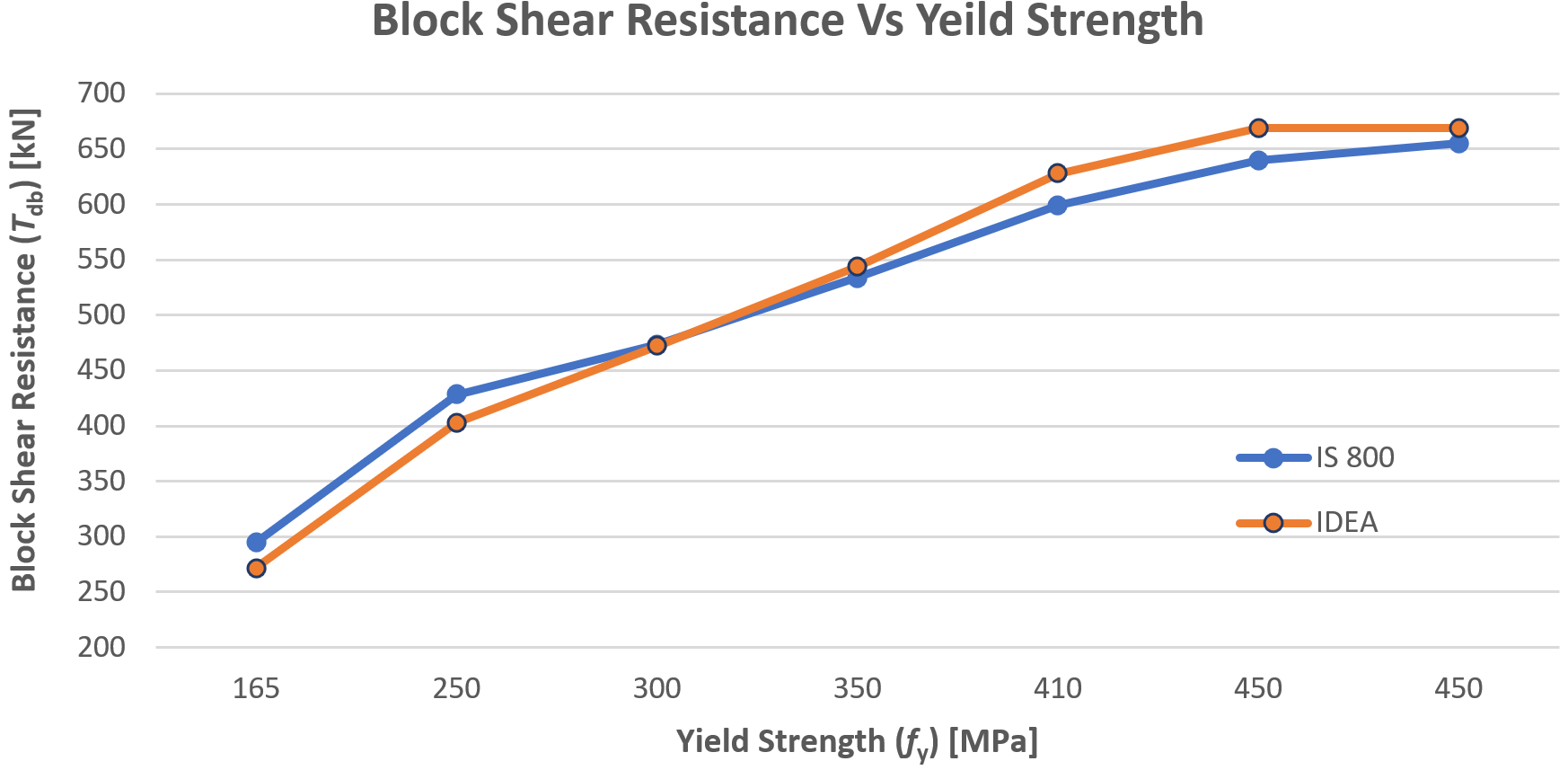Sample files Open in Viewer Download$$